Courses

# Flow Through Branched Pipes (Part - 1) Civil Engineering (CE) Notes | EduRev

## Mechanical Engineering : Flow Through Branched Pipes (Part - 1) Civil Engineering (CE) Notes | EduRev

The document Flow Through Branched Pipes (Part - 1) Civil Engineering (CE) Notes | EduRev is a part of the Mechanical Engineering Course Fluid Mechanics - Notes, Videos, MCQs & PPTs.
All you need of Mechanical Engineering at this link: Mechanical Engineering

## Flow Through Branched Pipes

In several practical situations, flow takes place under a given head through different pipes jointed together either in series or in parallel or in a combination of both of them.

## Pipes in Series

• If a pipeline is joined to one or more pipelines in continuation, these are said to constitute pipes in series. A typical example of pipes in series is shown in Fig. 36.1. Here three pipes A, B and are joined in series.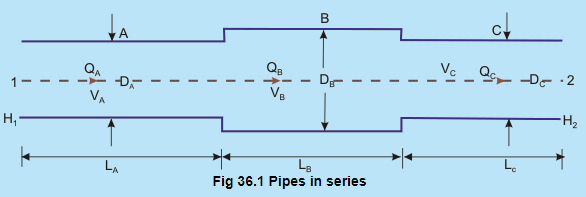n this case, rate of flow remains same in each pipe. Hence,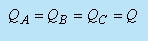If the total head available at Sec. 1 (at the inlet to pipe A) is  Hwhich is greater than H2 ,    the total head at Sec. 2 (at the exit of pipe C), then the flow takes place from 1 to 2 through the system of pipelines in series.

• Application of Bernoulli's equation between Secs.1 and 2 gives

H1 - H =   hf

where,  his the loss of head due to the flow from 1 to 2. Recognizing the minor and major losses associated with the flow, h can be written as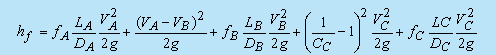(36.1)

Friction loss Loss due to
enlargement at
entry to pipe B
in pipe AFriction loss
in pipe B

The subscripts A, B and refer to the quantities in pipe A, B and C respectively. Cc is the coefficient of contraction.

• The flow rate Q satisfies the equation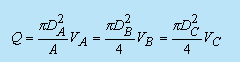Velocities VA, VB and VC in Eq. (36.1) are substituted from Eq. (36.2), and we get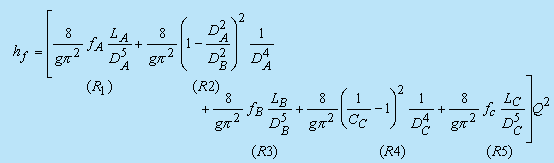(36.3)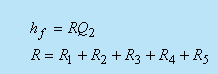(36.4)

Equation (36.4) states that the total flow resistance is equal to the sum of the different resistance components. Therefore, the above problem can be described by an equivalent electrical network system as shown in Fig. 36.2.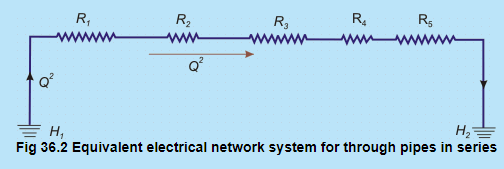Offer running on EduRev: Apply code STAYHOME200 to get INR 200 off on our premium plan EduRev Infinity!

## Fluid Mechanics - Notes, Videos, MCQs & PPTs

52 videos|76 docs|45 tests

,

,

,

,

,

,

,

,

,

,

,

,

,

,

,

,

,

,

,

,

,

;